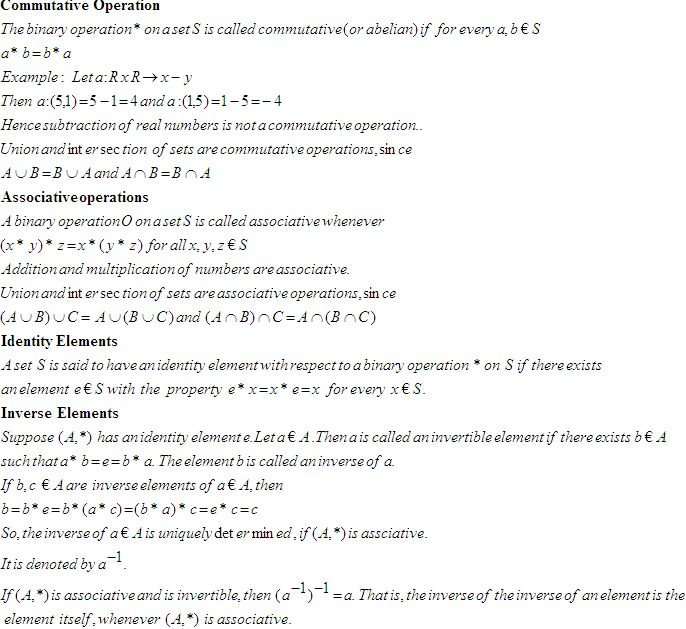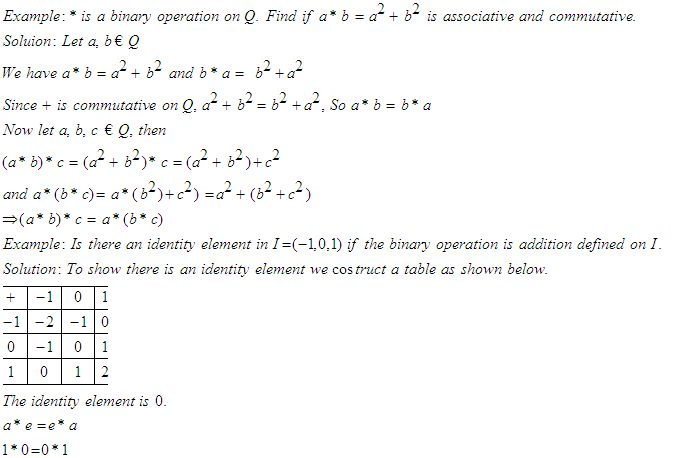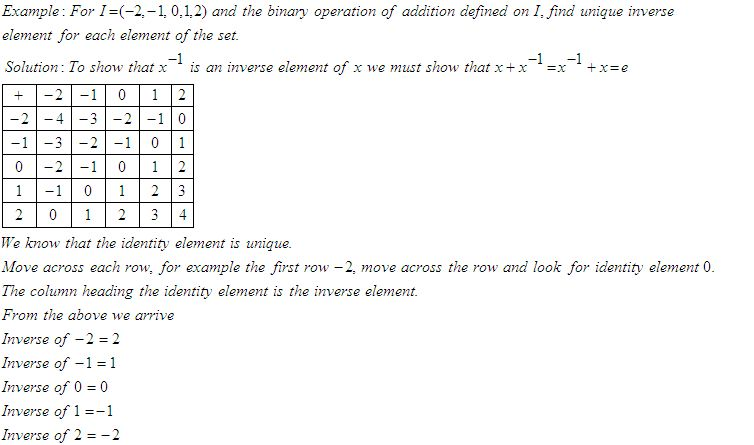Name: ___________________Date:___________________

 Email us to get an instant 20% discount on highly effective K-12 Math & English kwizNET Programs!

### High School Mathematics - 25.2 Laws of Binary OperationsDirections: Answer the following.
 Q 1: Find the identity element on a*b = a+ab, a, b Ć 1Answer: Q 2: Let * be a binary operation defined on Q. Check if a*b = a-b, for a,b Ć Q is commutative.YesNo Q 3: Let a*b = l.c.m of (a,b), a, b Ć N, find 3*512158 Q 4: Let a * b be a binary operation on N given by a * b = l.c.m (a,b), a, b, Ć N, find 2*4824 Q 5: Let A = N x N and let * be a binary operation on A defined by (a,b)*(c,d) = (ac, bd). Show that (A,*) is associative.Answer: Q 6: Let * be a binary operation defined on Q. Find if a*b = ab/4 , for a,b Ć Q is associative.Answer: Q 7: Find the identity element if a*b = a+b+ab , a, b Ć 1Answer: Q 8: Let * be a binary operation defined on Q. Check if a*b = a2+b2, for a,b Ć Q is commutative.NoYes Question 9: This question is available to subscribers only! Question 10: This question is available to subscribers only!